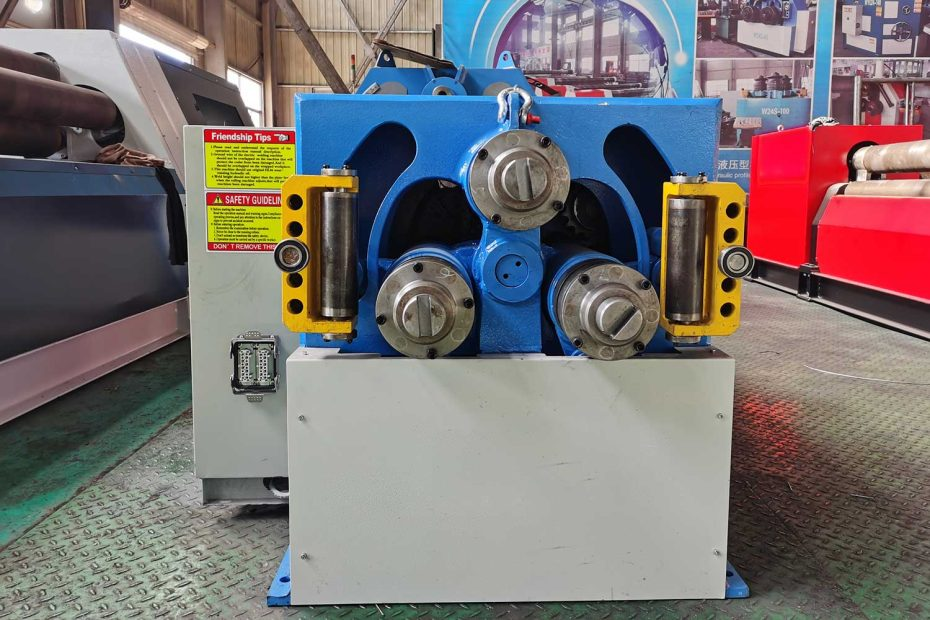# Demystifying Section Bending Stiffness: A Comprehensive ExplorationWhen it comes to understanding the mechanics of structures, section bending stiffness is a crucial concept that plays a pivotal role. It lies at the heart of how materials and shapes respond to external forces, ultimately determining the integrity and performance of everything from bridges and buildings to industrial machinery.

## The Basics of Section Bending Stiffness

• Definition: Section bending stiffness, often referred to simply as bending stiffness, is a property that quantifies how resistant a material or structural element is to bending under an applied load. It measures the material’s ability to resist deformation and maintain its shape when subjected to bending forces.
• Mathematical Representation: Bending stiffness is represented by the symbol “EI” in mathematical equations. Here, “E” stands for the modulus of elasticity, a material property that describes its stiffness, and “I” represents the second moment of area, which characterizes the cross-sectional shape’s resistance to bending.
• Units of Measurement: The units for bending stiffness are typically Newton meters squared (Nm²) or pound-force inches squared (lb·in²), depending on the unit system being used.

## Understanding the Significance of Bending Stiffness

Bending stiffness is a fundamental concept in structural engineering and mechanics. It has significant implications for a wide range of applications, including:

1. Structural Stability: Bending stiffness is a critical factor in determining the stability and load-bearing capacity of structures. It helps prevent excessive deflection and ensures that a structure can safely support its intended loads without failure.
2. Design Optimization: Engineers use bending stiffness to optimize the design of beams, columns, and other structural elements. By selecting materials and cross-sectional shapes with appropriate bending stiffness values, they can achieve the desired performance and efficiency.
3. Material Selection: Different materials have varying levels of bending stiffness. Engineers must consider this property when choosing materials for specific applications. For example, a material with high bending stiffness may be preferred for a rigid structure, while a material with lower bending stiffness may be suitable for a flexible component.
4. Dynamic Analysis: Bending stiffness is crucial in dynamic analysis, such as when assessing the response of a structure to vibrations or oscillations. It influences the natural frequencies and mode shapes of structures, which are essential for ensuring stability and avoiding resonance.

List of Key Factors Influencing Bending Stiffness:

• Cross-sectional shape
• Material properties (modulus of elasticity)
• Geometric dimensions